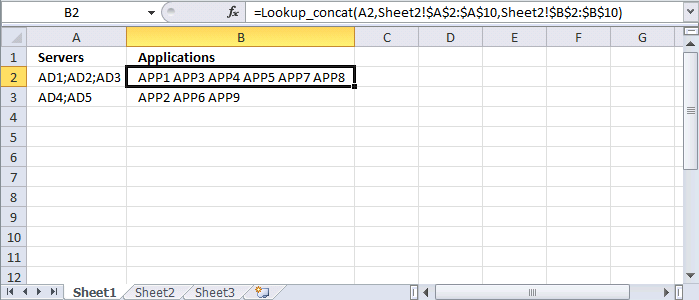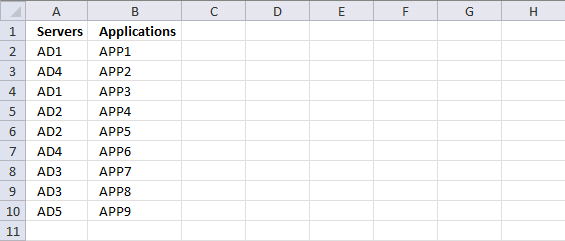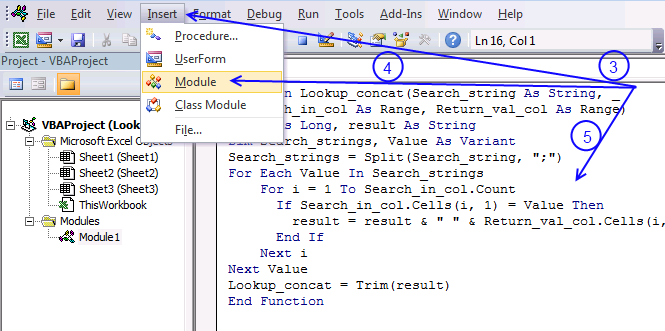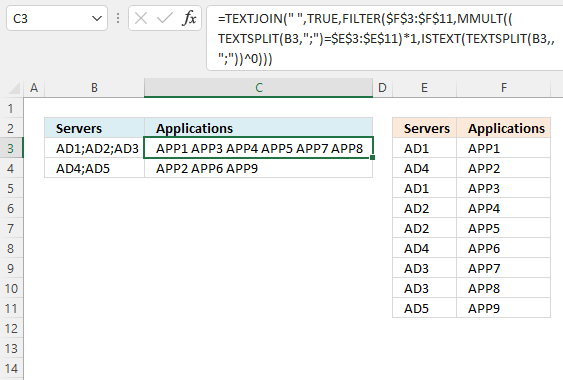Author: Oscar Cronquist Article last updated on August 15, 2022This article explains how to perform multiple lookups based on values in one cell with a delimiting character using a User defined Function or an Excel 365 dynamic array formula.

## 1. Lookup multiple values in one cell [UDF]A UDF (User defined Function) is an Excel function that you can build yourself which is great if you can't find a prebuilt one that suits your needs.

You create a UDF the same way you create a macro using VBA code in the VB Editor (Visual Basic Editor), VBA stands for Visual Basic for Applications.

I will explain in greater detail the code I used, where to put it, and how to save your workbook,  later in this article.

Hi Oscar
This is a very interesting function and helped me a lot so far. My file though is a bit more complicated.

I have multiple info in one cell separated with ";" (example AD1; AD2; AD3) let's say that these are servers (File name SERVERS) and in each server, I have multiple applications.

I have now another file that has all the applications per server per line in excel (each line has one server one application. Filename: APPS).

I want to start from the file SERVERS to look up the servers that are in one cell find them in the second file APPS and bring all the applications also in one cell in the file SERVERS.

Any ideas here?
CWorksheet Sheet2 contains the items and the corresponding applications.Worksheet Sheet1 contains the concatenated items in column A and a formula in column B. The first argument in the UDF is the cell that contains the concatenated values, this cell reference is relative meaning it changes when you copy cell B2 and paste it to cells below.

The second argument is the lookup range and the third argument is the return range, both these arguments have absolute cell references.

Lookup_concat(Search_string,  Search_in_col , Return_val_col )

Formula in cell B2:

=Lookup_concat(A2, Sheet2!\$A\$2:\$A\$10, Sheet2!\$B\$2:\$B\$10)

### VBA code

```'Name the UDF and declare arguments and data types
Function Lookup_concat(Search_string As String, _
Search_in_col As Range, Return_val_col As Range)

'Dimension variables and declare data types
Dim i As Long, result As String
Dim Search_strings, Value As Variant

'Split string using a delimiting character and return an array of values
Search_strings = Split(Search_string, ";")

'Iterate through values in array
For Each Value In Search_strings

'Iterate through from 1 to the number of cells in Search_in_col
For i = 1 To Search_in_col.Count

'Check if cell value is equal to value in variable Value
If Search_in_col.Cells(i, 1) = Value Then

'Save the corresponding return value to variable result
result = result & " " & Return_val_col.Cells(i, 1).Value
End If

'Continue with next number
Next i

'Continue with next value
Next Value

'Return values saved to result to worksheet
Lookup_concat = Trim(result)
End Function
```

### Where to put the code?You can use the code (see instructions below) in your workbook or get the example file.

1. Copy VBA code above.
2. Open VB Editor (Alt+F11)  and select your workbook in the Project Explorer.
3. Press with left mouse button on Insert on the menu.
4. Press with left mouse button on Module to create a module.
5. Paste code to module1
6. Exit VB Editor

Note, save your workbook with file extension *.xlsm (macro enabled) to make sure you attach the code to your workbook.

### Get the Excel fileLookup-multiple-values-in-one-cell.xlsm

## 2. Lookup multiple values in one cell - Excel 365This Excel 365 formula splits a given cell value based on a specific delimiter and performs a lookup for each substring, then the corresponding value on the same row is returned and concatenated together with the remaining results.

Excel 365 formula in cell C3:

=TEXTJOIN(" ", TRUE, FILTER(\$F\$3:\$F\$11, MMULT((TEXTSPLIT(B3, ";")=\$E\$3:\$E\$11)*1, ISTEXT(TEXTSPLIT(B3, , ";"))^0)))

### Explaining formula

#### Step 1 - Split cell value

The TEXTSPLIT function splits a string into an array based on delimiting values.

Function syntax: TEXTSPLIT(Input_Text, col_delimiter, [row_delimiter], [Ignore_Empty])

TEXTSPLIT(B3, ";")

becomes

and returns

#### Step 2 - Compare substrings to a lookup table

The equal sign lets you compare value to value, you can also compare array to array as long as the first array is horizontal and the second array is vertical or vice versa.

TEXTSPLIT(B3, ";")=\$E\$3:\$E\$11

becomes

and returns

{TRUE,FALSE,FALSE ; FALSE,FALSE,FALSE ; TRUE,FALSE,FALSE ; FALSE,TRUE,FALSE ; FALSE,TRUE,FALSE ; FALSE,FALSE,FALSE ; FALSE,FALSE,TRUE ; FALSE,FALSE,TRUE ; FALSE,FALSE,FALSE}

#### Step 3 - Convert boolean values

Step 2 returned an array of boolean values, the MMULT function can't handle boolean values. The asterisk character lets you multiply values in an Excel formula, it is also possible to multiply boolean values and in the process create their numerical equivalents.

TRUE equals 1 and FALSE equals 0 (zero).

(TEXTSPLIT(B3, ";")=\$E\$3:\$E\$11)*1

becomes

{TRUE,FALSE,FALSE ; FALSE,FALSE,FALSE ; TRUE,FALSE,FALSE ; FALSE,TRUE,FALSE ; FALSE,TRUE,FALSE ; FALSE,FALSE,FALSE ; FALSE,FALSE,TRUE ; FALSE,FALSE,TRUE ; FALSE,FALSE,FALSE}*1

and returns

{1,0,0 ; 0,0,0 ; 1,0,0 ; 0,1,0 ; 0,1,0 ; 0,0,0 ; 0,0,1 ; 0,0,1 ; 0,0,0}.

#### Step 4 - Create an array

We need an array of numbers that match the number of substrings found in the cell, they all need to be 1 so the MMULT function can calculate properly.

The ISTEXT function returns TRUE if argument is text.

Function syntax: ISTEXT(value)

ISTEXT(TEXTSPLIT(B3,,";"))

becomes

and returns

{TRUE; TRUE; TRUE}.

#### Step 5 - Convert boolean values

The power of character ^ lets you convert the boolean value to 1 if you calculate the power of 0 (zero).

ISTEXT(TEXTSPLIT(B3, , ";"))^0

becomes

{TRUE; TRUE; TRUE}^0

and returns {1; 1; 1}.

#### Step 6 - Sum numbers row-wise

The MMULT function calculates the matrix product of two arrays, an array as the same number of rows as array1 and columns as array2.

Function syntax: MMULT(array1, array2)

MMULT((TEXTSPLIT(B3, ";")=\$E\$3:\$E\$11)*1, ISTEXT(TEXTSPLIT(B3, , ";"))^0)

becomes

MMULT({1,0,0 ; 0,0,0 ; 1,0,0 ; 0,1,0 ; 0,1,0 ; 0,0,0 ; 0,0,1 ; 0,0,1 ; 0,0,0},{1; 1; 1})

and returns

{1; 0; 1; 1; 1; 0; 1; 1; 0}.

#### Step 7 - Filter values based on array

The FILTER function extracts values/rows based on a condition or criteria.

Function syntax: FILTER(array, include, [if_empty])

FILTER(\$F\$3:\$F\$11, MMULT((TEXTSPLIT(B3, ";")=\$E\$3:\$E\$11)*1, ISTEXT(TEXTSPLIT(B3, , ";"))^0))

becomes

FILTER({"APP1";"APP2";"APP3";"APP4";"APP5";"APP6";"APP7";"APP8";"APP9"}, {1; 0; 1; 1; 1; 0; 1; 1; 0})

and returns

{"APP1"; "APP3"; "APP4"; "APP5"; "APP7"; "APP8"}.

#### Step 8 - Concatenate values

The TEXTJOIN function combines text strings from multiple cell ranges.

Function syntax: TEXTJOIN(delimiter, ignore_empty, text1, [text2], ...)

TEXTJOIN(" ", TRUE, FILTER(\$F\$3:\$F\$11, MMULT((TEXTSPLIT(B3, ";")=\$E\$3:\$E\$11)*1, ISTEXT(TEXTSPLIT(B3, , ";"))^0)))

becomes

TEXTJOIN(" ", TRUE, {"APP1"; "APP3"; "APP4"; "APP5"; "APP7"; "APP8"})

and returns

"APP1 APP3 APP4 APP5 APP7 APP8".